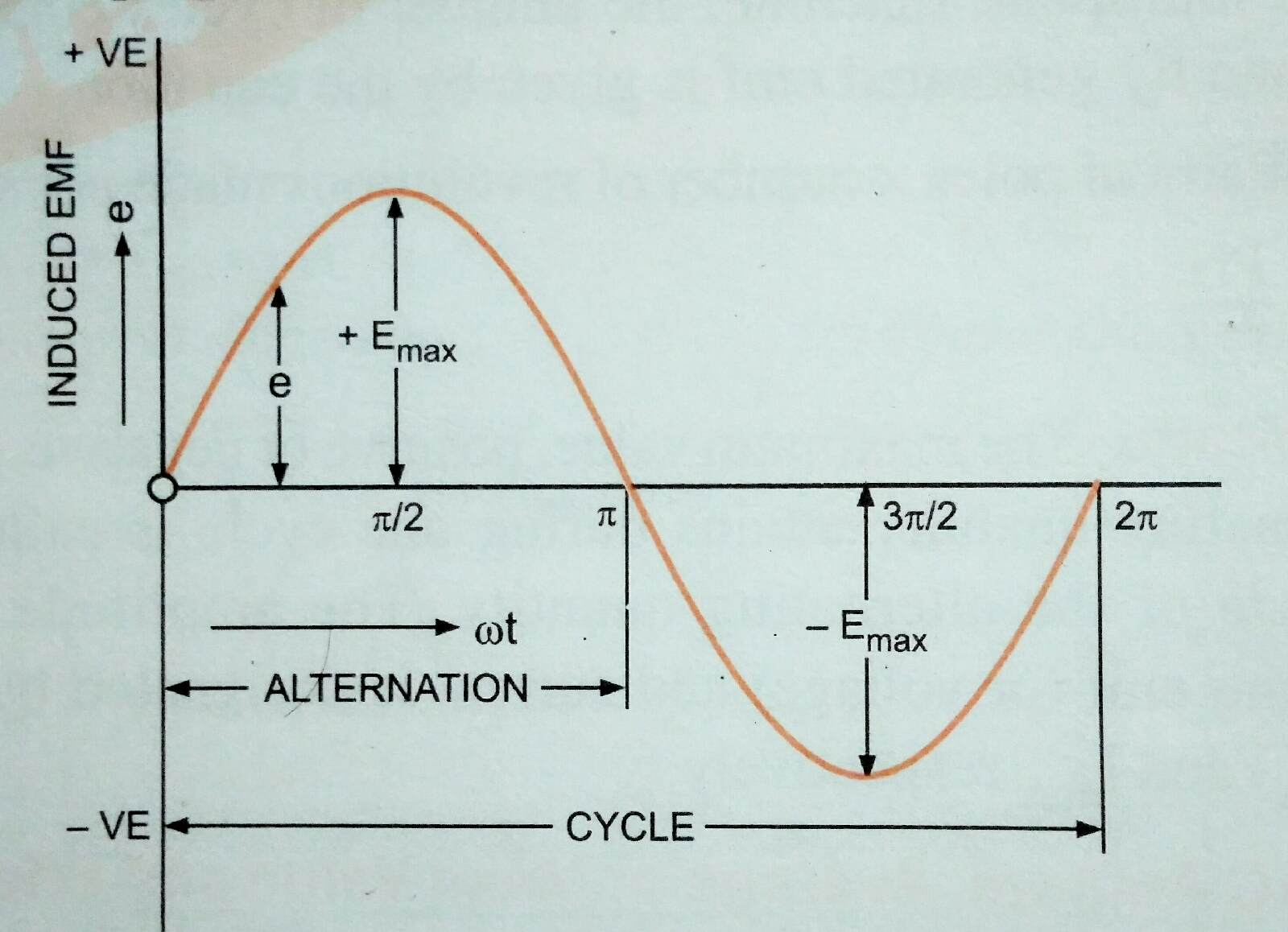### Ticker

6/recent/ticker-posts

# AC BASIC AND NETWORKS

FUNDAMENTALS OF AC

Alternative Quantity

--------> A current or Voltage is called alternating if it is reverses periodically in direction and it's magnitude undergoes  a difined cycle of changes in definite intervals of time. Each cycle of alternating current or Voltage consists of two half cycles, during one of which the Current or Voltage acts in one direction, while during the other in opposite direction. In more restricted sense alternating current is a periodically varying Current the average value of which over a period is zero. At present a large percentage of the Electrical energy (nearly all) being used for domestic and commercial purposes is generated an alternating Current. In fact almost the whole of the vast amount of Electrical Energy used through out the world for every imaginable purpose is genenrated by alternating current genenrators. The reasons for genenration of Electrical energy in form of AC are: low construction and operating cost per KW. Economical transmission and distribution high transmission and efficiency, simpler switch gear in case of AC system law Maintenance cost of AC equipments etc.

Generation of alternating voltages and cutrents.

An alternating EMF can be generated eighter by rotating a coil within a stationary magnetic field or by rotating magnetic field within a stationary coil. The magnitude of EMF generated in the coil depend upon the number of turnes on coil strength of megnetic feild and the speed at which the coil or megnetic field rotates.

Sinusoidal Quantities [ EMF , VOLTAGE AND CURRENT]

It is not an accident that the bulk of electric power generated in Electric power station through out the world and distributed to the consumer appears in the form of sinusoidal variation of voltage and current. There are Many Technical and economical advantages associated with the use of sinusoidal voltage and currents.
In practical Electrical Engineering it is assumed to that the alternating voltages and currents are sinusoidal through to They may slightly devited From it. The advantage of this assumption in that calculation become simple.
It may be noted alternating voltage and current mean sinusoidal Voltage and Current unless stated otherwise.
Alternating EMF following sine law ( i.e sinusoidal emf ) is illustrated in fig. And is expressed in the formSinusoidal Emf

e   =  E max  sin 2πft
Where e is the instantaneous value of alternating EMF (or Voltage) Emax is thea maximum value of the alternating EMF and 2πf is angular velocity of the coil.

The rotating coil moves through an angle or 2π radians in one cycle, so angular velocity w = 2πf where f is the number of Cycle complete per second.

Substituting w = 2πf
e =  Emax sin 2πft
If the alternating EMF given by w =2πft
Is applied across a load alternating current flows through the Circuit which would also vary sinusoidal i.e following a sine law . The expression for alternating Current is given as :

i = Imax sin 2πft = Imax  sin 2πft.